## Eigenvalues and Eigenvectors

Consider multiplying a square 3x3 matrix by a 3x1 (column) vector. The result is a 3x1 (column) vector. The 3x3 matrix can be thought of as an operator - it takes a vector, operates on it, and returns a new vector. There are many instances in mathematics and physics in which we are interested in which vectors are left "essentially unchanged" by the operation of the matrix. Specifically, we are interested in those vectors v for which Av=kv where A is a square matrix and k is a real number. A vector v for which this equation hold is called an eigenvector of the matrix A and the associated constant k is called the eigenvalue (or characteristic value) of the vector v. If a matrix has more than one eigenvector the associated eigenvalues can be different for the different eigenvectors.

Geometrically, the action of a matrix on one of its eigenvectors causes the vector to stretch (or shrink) and/or reverse direction.

In order to find the eigenvalues of a nxn matrix A (if any), we solve Av=kv for scalar(s) k. Rearranging, we have Av-kv=0. But kv=kIv where I is the nxn identity matrix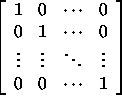So, 0=Av-kv=Av-kIv=(A-kI)v. This equation is equivalent to a homogeneous system of n equations with n unknowns. This equation has a non-zero solution for v if and only if the determinant det(A-kI) is zero. Thus, by finding the zeros of the polynomial in k determined by the characteristic equation det(A-kI)=0, we will have found the eigenvalues of the matrix A.

#### Example

To find the eigenvalues of the matrix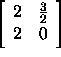we substitute A into the equation det(A-kI)=0 and solve for k. The matrix A-kI is given by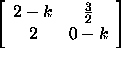which has determinant k^2-2k-3. Hence, we are looking for values k satisfying k^2-2k-3=0. So, of course, we have k=3 or k=-1 . To find the eigenvectors of the eigenvalue k=3 we look for solutions v of the homogeneous system of equations (A-3I)v=0: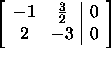Since the second equation is a constant multiple of the first, this system of equations reduces to the single equation -x+(3/2)y=0 or equivalently x=1.5y. This system has an infinite number of solutions. So for example, choosing y=2 yeilds the vector <3,2> which is thus an eigenvector that has eigenvalue k=3. In a general form, all eigenvectors with eigenvalue 3 have the form <2t,3t> where t is any real number. It can also be shown (by solving the system (A+I)v=0) that vectors of the form <t,-2t> are eigenvectors with eigenvalue k=-1.

#### Example

Find the eigenvalues and corresponding eigenvalues for the matrix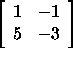First, we must find det(A-kI):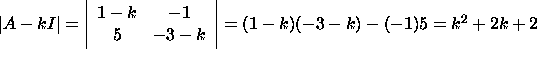This leads to the characteristic equation k^2+2k+2=0 which has complex roots k=-1+i and k=-1-i. To find the eigenvectors for k=-1+i, we solve (A-(-1+i)I)v=0 for v: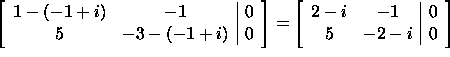The second equation is a constant multiple of the first equation so the system reduces to the single equation (2-i)x-y=0 which implies y=(2-i)x. There are once again an infinite number of eigenvectors of A of the form <t,(2-i)t> with eigenvalue k=-1+i. By examining the system of equations (A-(-1-i)I)v=0 it can also be shown that vectors of the form <t,(2+i)t> are eigenvectors of A with eigenvalue k=-1-i.

From the examples above we can infer a property of eigenvectors and eigenvalues: eigenvectors from distinct eigenvalues are linearly independent. The following examples illustrate that the situation is not so clear cut when the eigenvalues are not distinct.

#### Example

The matrix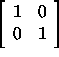has two eigenvalues (1 and 1) but they are obviously not distinct. Since A is the identity matrix, Av=v for any vector v, i.e. any vector is an eigenvector of A. We can thus find two linearly independent eigenvectors (say <-2,1> and <3,-2>) one for each eigenvalue.

#### Example

The matrix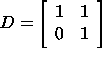also has non-distinct eigenvalues of 1 and 1. All eigenvalues are solutions of (A-I)v=0 and are thus of the form <t,0>. Hence, in this case there do not exist two linearly independent eigenvectors for the two eigenvalues 1 and 1 since <t,0> and <s,0> are not linearly independent for any values of s and t.

#### Symmetric Matrices

There is a very important class of matrices called symmetric matrices that have quite nice properties concerning eigenvalues and eigenvectors. A symmetric matrix A is a square matrix with the property that A_ij=A_ji for all i and j. The matrices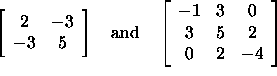are symmetric matrices. In symmetric matrices the upper right half and the lower left half of the matrix are mirror images of each other about the diagonal.

A nxn symmetric matrix A not only has a nice structure, but it also satisfies the following:

1. A has exactly n (not necessarily distinct) eigenvalues
2. There exists a set of n eigenvectors, one for each eigenvalue, that are mututally orthogonal.

Thus, the situation encountered with the matrix D in the example above cannot happen with a symmetric matrix: A symmetric matrix has n eigenvalues and there exist n linearly independent eigenvectors (because of orthogonality) even if the eigenvalues are not distinct.

#### Example

Find the eigenvalues and a set of mutually orthogonal eigenvectors of the symmetric matrixFirst we need det(A-kI):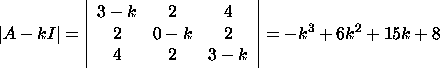Thus, the characteristic equation is (k-8)(k+1)^2=0 which has roots k=-1, k=-1, and k=8. Note that we have listed k=-1 twice since it is a double root. We must find two eigenvectors for k=-1 and one for k=8. We now examine (A+I)v=0 to find the eigenvectors for the eigenvalue k=-1: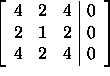It is easily seen that this system reduces to the single equation 2x+y+2z=0 since the other two equations are twice this one. There are two parameters here (x and z); thus, eigenvectors for k=-1 must have the form y=-2x-2z which corresponds to vectors of the form <s,-2s-2t,t>. We must choose values of s and t that yield two orthogonal vectors (the third comes from the eigenvalue k=8). First, choose anything, say s=1 and t=0: <1,-2,0>. Now find a vector <x,-2x-2z,z> such that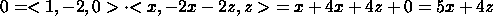An easy choice here is x=4 and z=-5. So, we now have two orthogonal vectors <1,-2,0> and <4,2,-5> that correspond to the two instances of the eigenvalue k=-1. It can also be shown that the eigenvectors for k=8 are of the form <2r,r,2r> for any value of r. It is easy to check that this vector is orthogonal to the other two we have for any choice of r. So, let's take r=1. We now have the following: eigenvalues and orthogonal eigenvectors:

for k=-1: <1,-2,0> and <4,2,-5>

for k=8: <2,1,2>

Note that since this matrix is symmetric we do indeed have 3 eigenvalues and a set of 3 orthogonal (and thus linearly independent) eigenvectors (one for each eigenvalue).